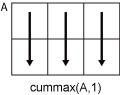Documentation

cummax

Cumulative maximum

Description

example

M = cummax(A) returns the cumulative maximum elements of A. By default, cummax(A) operates along the first array dimension whose size does not equal 1.

• If A is a vector, then cummax(A) returns a vector of the same size containing the cumulative maxima of A.

• If A is a matrix, then cummax(A) returns a matrix of the same size containing the cumulative maxima in each column of A.

• If A is a multidimensional array, then cummax(A) returns an array of the same size containing the cumulative maxima along the first array dimension of A whose size does not equal 1.

example

M = cummax(A,dim) returns the cumulative maxima along the dimension dim. For example, if A is a matrix, then cummax(A,2) returns the cumulative maxima along the rows of A.

example

M = cummax(___,direction) optionally specifies the direction using any of the previous syntaxes. You must specify A and, optionally, can specify dim. For instance, cummax(A,2,'reverse') returns the cumulative maxima of A by working from end to beginning of the second dimension of A.

example

M = cummax(___,nanflag) specifies whether to include or omit NaN values from the calculation for any of the previous syntaxes. cummax(A,'includenan') includes all NaN values in the calculation while cummax(A,'omitnan') ignores them.

Examples

collapse all

Find the cumulative maxima of a 1-by-10 vector of random integers.

v = randi(10,1,10)
v = 1×10

9    10     2    10     7     1     3     6    10    10

M = cummax(v)
M = 1×10

9    10    10    10    10    10    10    10    10    10

Find the cumulative maxima of the columns of a 3-by-3 matrix.

A = [3 5 2; 1 6 3; 7 8 1]
A = 3×3

3     5     2
1     6     3
7     8     1

M = cummax(A)
M = 3×3

3     5     2
3     6     3
7     8     3

Find the cumulative maxima of the rows of a 3-by-3 matrix.

A = [3 5 2; 1 6 3; 7 8 1]
A = 3×3

3     5     2
1     6     3
7     8     1

M = cummax(A,2)
M = 3×3

3     5     5
1     6     6
7     8     8

Calculate the cumulative maxima in the third dimension of a 2-by-2-by-3 array. Specify direction as 'reverse' to work from the end of the third dimension to the beginning.

A = cat(3,[1 2; 3 4],[9 10; 11 12],[5 6; 7 8])
A =
A(:,:,1) =

1     2
3     4

A(:,:,2) =

9    10
11    12

A(:,:,3) =

5     6
7     8

M = cummax(A,3,'reverse')
M =
M(:,:,1) =

9    10
11    12

M(:,:,2) =

9    10
11    12

M(:,:,3) =

5     6
7     8

Create a vector containing NaN values and compute the cumulative maxima. By default, cummax ignores NaN values.

A = [3 5 NaN 9 0 NaN];
M = cummax(A)
M = 1×6

3     5     5     9     9     9

If you include NaN values in the calculation, then the cumulative maximum becomes NaN as soon as the first NaN value in A is encountered.

M = cummax(A,'includenan')
M = 1×6

3     5   NaN   NaN   NaN   NaN

Input Arguments

collapse all

Input array, specified as a vector, matrix, or multidimensional array. For complex elements, cummax compares the magnitude of the elements. If magnitudes are equal, cummax also compares the phase angles.

Data Types: single | double | int8 | int16 | int32 | int64 | uint8 | uint16 | uint32 | uint64 | logical | duration
Complex Number Support: Yes

Dimension to operate along, specified as a positive integer scalar. If no value is specified, then the default is the first array dimension whose size does not equal 1.

Consider a two-dimensional input array, A:

• cummax(A,1) works on successive elements in the columns of A and returns an array of the same size as A with the cumulative maxima in each column.• cummax(A,2) works on successive elements in the rows of A and returns an array of the same size as A with the cumulative maxima in each row.cummax returns A if dim is greater than ndims(A).

Direction of cumulation, specified as 'forward' (default) or 'reverse'.

• 'forward' works from 1 to end of the active dimension.

• 'reverse' works from end to 1 of the active dimension.

Data Types: char

NaN condition, specified as one of the following values:

• 'omitnan' — Ignore all NaN values in the input. If the input has consecutive leading NaN values, then cummax returns NaN in the corresponding elements of the output. For example, cummax([NaN 7 13 6],'omitnan') returns the row vector [NaN 7 13 13].

• 'includenan' — Include NaN values from the input when computing the cumulative maxima, resulting in NaN values in the output.

Data Types: char

Output Arguments

collapse all

Cumulative maxima, returned as a vector, matrix, or multidimensional array. The size and data type of M are the same as those of A.

Tips

• The 'reverse' option in many cumulative functions allows quick directional calculations without requiring a flip or reflection of the input array.